Feature Articles: A New Era in Quantum Information Processing Technologies

# Quantum Neural Network for Solving Complex Combinatorial Optimization Problems

## Abstract

Optimization of various social systems, including communication, traffic, and social networking systems, requires the solving of complex combinatorial optimization problems. In this article, we describe the recent progress achieved in developing a quantum neural network, a computer that finds solutions to combinatorial optimization problems using a network of degenerate optical parametric oscillators.

Keywords: Ising model, combinatorial optimization problem, optical parametric oscillator

## 1. Introduction

As various systems in our society grow larger and more complex, it becomes increasingly important to analyze and optimize such systems. These complex problems are classified as combinatorial optimization problems, which cannot be solved efficiently with conventional digital computers.

It is known that such problems can be converted to ground-state-search problems of the Ising model, which is a theoretical model proposed by E. Ising that describes a group of interacting spins. Several institutions recently reported artificial spin networks that were applied to simulate the Ising model in order to solve combinatorial optimization problems [1, 2]. Our team has been studying such an Ising-type computer called a quantum neural network (QNN) . In a QNN, Ising spins are represented by degenerate optical parametric oscillators (DOPOs), which are coupled with mutual injections of DOPO light. In this article, we describe the basic working principle of a QNN and report the recent experimental progress made in this area at NTT.

## 2. Ising model

The Hamiltonian of the Ising model is given as follows.

H = − Σi<jJijσiσj. 　　　　　　　　　　　　　　　(1)

Here, σi and Jij respectively represent the ith spin state and the coupling coefficient between the ith and jth spins. The ground state of the Ising model corresponds to a set of spins {σi} that minimize Eq. (1) for a given matrix {Jij}. A QNN, also known as a coherent Ising machine, is a network of artificial spins based on quantum optical oscillators that simulates the Ising model . In our QNN, a spin state is represented by a DOPO, and the spin-spin interaction coefficient Jij is implemented by adjusting the length and transmittance of an optical path between the ith and jth DOPO (Fig. 1). Since the networked DOPO tends to oscillate with a phase configuration that minimizes the total energy, we can obtain the ground state of a given Ising model by simply reading the phases of the DOPOs that are above the threshold.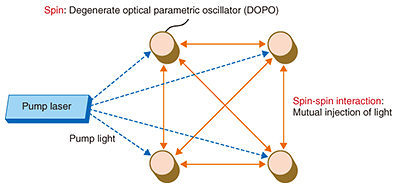Fig. 1. Concept of quantum neural network (QNN).

## 3. Phase sensitive amplification

An important physical phenomenon for generating a DOPO is a nonlinear optical effect called phase sensitive amplification . When we input a pump light (angular frequency ωp) and a signal light ωs to a medium with the second or third order optical nonlinearity, we can generate an idler light with an angular frequency ωi = ωpωs (second order case) and an initial phase −θ, where θ represents the initial phase difference between the pump and the signal. In particular, when the signal and idler frequencies degenerate (ωs = ωi), the amplification coefficient of the signal amplitude is proportional to cosθ, which means that the light is efficiently amplified when its initial phase difference from the pump is either 0 or π.

To achieve such a phase sensitive amplifier (PSA), we can utilize four-wave mixing in an optical fiber [5, 6] or parametric down-conversion in a periodically poled lithium niobate waveguide [4, 7]. If we pump a PSA placed in an optical cavity, the 0- or π-phase component of the spontaneous emission noise is most efficiently amplified by the PSA, which eventually leads to an optical parametric oscillation with either one of the binary phase states (Fig. 2). In addition, if we use a pulsed pump whose temporal interval is set at 1/N of the cavity round-trip time, we can generate N time-multiplexed DOPOs using a single cavity.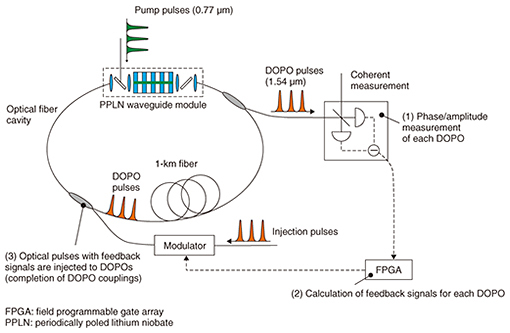Fig. 2. QNN configuration.

We succeeded in generating more than thousands of DOPOs using a 1-km fiber cavity, paving the way towards achieving a large-scale QNN [5–7]. More recently, we reported the generation of more than 1 million DOPOs using a 10-GHz clock pump and a 20-km fiber cavity .

We can implement couplings between time-multiplexed DOPOs by inserting delayed interferometers. In an experiment reported in an earlier paper , we implemented nearest-neighbor coupling between DOPOs by placing a 1-bit delayed interferometer in the fiber cavity so that we could realize a one-dimensional Ising model. With the optically coupled DOPOs, we successfully observed that the DOPOs showed ferro- and antiferromagnetic behavior when we changed the phase difference between the two arms of the interferometer, suggesting that the DOPOs well simulated the characteristics of low-temperature spins.

## 4. Measurement-feedback scheme

The above one-dimensional Ising model experiment was important for understanding the physics of a QNN. However, it is difficult to solve real-world combinatorial optimization problems that are usually represented by large and complex spin networks using an optically coupled DOPO network. For example, in order to achieve all-to-all connection between 2000 DOPOs, we need as many as 1999 interferometers placed in the cavity, which is very hard to do experimentally.

We employed the measurement-feedback scheme shown in Fig. 2 in order to achieve flexible couplings among thousands of DOPOs. In this scheme, we measure the amplitudes of all N DOPOs {ci} while the DOPOs are circulating in the cavity and while controlling the evolution of their amplitudes. The measurement results are fed to a field programmable gate array (FPGA), where the spin-spin coupling matrix {Jij} for a given problem is set in advance. In each DOPO circulation in the cavity, the FPGA calculates si = ΣjJijcj, the feedback signal for the ith DOPO in the next round trip. The feedback signal si is used to modulate a light pulse whose frequency is exactly the same as that of the DOPOs, and the pulse is launched to the ith DOPO. As a result, any pair among N DOPOs can be coupled with this scheme. The number of such combinations is N(N−1)/2 when the problem is given by a directed graph.

We used the measurement-feedback scheme to construct a QNN with 2048 DOPOs , with which we succeeded in finding the solutions to maximum cut problems for a 2000-node graph (Fig. 3). In particular, when we applied the QNN to a maximum cut problem of a 2000-node complete graph, we obtained a solution that corresponds to a reference Ising energy in less than 100 µs, which is approximately 50 times shorter than the computation time obtained with simulated annealing run on a CPU (central processing unit) (Fig. 4).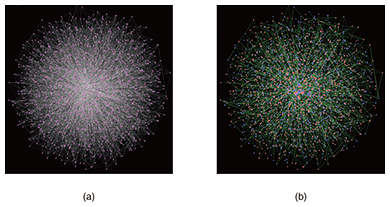Fig. 3. Solution searching of a 2000-node graph problem with QNN. (a) Graph problem (random graph with 19,990 edges). The pink dots and white lines respectively show the nodes and edges. (b) Solution obtained with the QNN experimentally. The pink dots are divided into red and blue dots; as a result, we can cut the edges shown by the green lines. Larger dots exhibit nodes with a larger number of edges.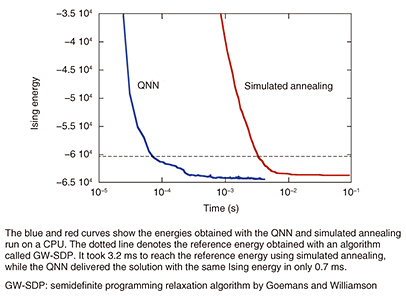Fig. 4. Ising energy as a function of computation time for a maximum cut problem of a 2000-node complete graph (K2000).

## 5. Future prospects

Although QNN research is still in a very early stage, our experimental results suggest that the QNN may outperform conventional digital computers for certain types of problems. We are currently planning to increase the number of DOPOs of our QNN to further widen the performance advantage over digital computers. We also plan to search for applications of QNN by collaborating with researchers from various fields such as statistical physics, mathematics, and computer science.

## References

 M. W. Johnson, M. H. S. Amin, S. Gildert, T. Lanting, F. Hamze, N. Dickson, R. Harris, A. J. Berkley, J. Johansson, P. Bunyk, E. M. Chapple, C. Enderud, J. P. Hilton, K. Karimi, E. Ladizinsky, N. Ladizinsky, T. Oh, I. Perminov, C. Rich, M. C. Thom, E. Tolkacheva, C. J. S. Truncik, S. Uchaikin, J. Wang, B. Wilson, and G. Rose, “Quantum Annealing with Manufactured Spins,” Nature, Vol. 473, pp. 194–198, 2011. M. Yamaoka, C. Yoshimura, M. Hayashi, T. Okuyama, H. Aoki, and H. Mizuno, “A 20k-spin Ising Chip to Solve Combinatorial Optimization Problems with CMOS Annealing,” IEEE J. Solid-State Circuits, Vol. 51, No. 1, pp. 303–309, 2015. S. Utsunomiya, K. Takata, and Y. Yamamoto, “Mapping of Ising Models onto Injection-locked Laser Systems,” Opt. Express, Vol. 19, No. 19, pp. 18091–18108, 2011. T. Umeki, O. Tadanaga, T. Kazama, K. Enbutsu, Y. Miyamoto, and H. Takenouchi, “Advances in Phase Sensitive Amplifiers Based on PPLN Waveguides for Optical Communication,” NTT Technical Review, Vol. 14, No. 1, 2016. https://www.ntt-review.jp/archive/ntttechnical.php?contents=ntr201601fa3.html H. Takesue, T. Inagaki, K. Inoue, and Y. Yamamoto, “Coherence Property of >2500-pulse Multiplexed Degenerate OPO,” The 61st JSAP (Japan Society of Applied Physics) Spring Meeting, 17p-PA1-1, Saga­mihara, Kanagawa, Japan, Mar. 2014 (in Japanese). H. Takesue, T. Inagaki, T. Umeki, O. Tadanaga, and H. Takenouchi, “Large-scale Time-division Multiplexed OPOs Using PPLN Waveguide,” The 62nd JSAP Spring Meeting, 12a-P6-1, Shonan, Kanagawa, Japan, Mar. 2015 (in Japanese). T. Inagaki, K. Inaba, R. Hamerly, K. Inoue, Y. Yamamoto, and H. Takesue, “Large-scale Ising Spin Network Based on Degenerate Optical Parametric Oscillators,” Nat. Photonics, Vol. 10, pp. 415–419, 2016. H. Takesue and T. Inagaki, “10 GHz Clock Time-multiplexed Degenerate Optical Parametric Oscillators for a Photonic Ising Spin Network,” Opt. Lett., Vol. 41, No. 18, pp. 4273–4276, 2016. T. Inagaki, Y. Haribara, K. Igarashi, T. Sonobe, S. Tamate, T. Honjo, A. Marandi, P. L. McMahon, T. Umeki, K. Enbutsu, O. Tadanaga, H. Takenouchi, K. Aihara, K. Kawarabayashi, K. Inoue, S. Utsunomiya, and H. Takesue, “A Coherent Ising Machine for 2000-node Optimization Problems,” Science, Vol. 354, No. 6312, pp. 603–606, 2016.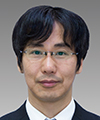Hiroki Takesue Senior Distinguished Scientist, Group Leader, Quantum Optical State Control Research Group, Optical Science Laboratory, NTT Basic Research Laboratories. He received a B.E., M.E., and Ph.D. in engineering science from Osaka University in 1994, 1996, and 2002. In 1996, he joined NTT Basic Research Laboratories, where he was engaged in research on lightwave frequency synthesis, optical access networks using wavelength division multiplexing, and quantum optics. He is currently pursuing research on communication and computation using quantum optics technologies. He is the recipient of several awards, including the ITU-T Kaleidoscope Conference 2nd Best Paper Award in 2008 and The Commendation for Science and Technology by the Minister of Education, Culture, Sports, Science and Technology of Japan (The Young Scientists’ Prize) in 2010. He was a visiting scholar at Stanford University, California, USA, from 2004 to 2005, and a guest researcher at the National Institute of Standards and Technology (NIST), Colorado, USA, in 2014. He is a Guest Professor in the Graduate School of Engineering Science, Osaka University, and a member of the Institute of Electrical and Electronics Engineers (IEEE) and the Japan Society of Applied Physics (JSAP). Takahiro Inagaki Researcher, Quantum Optical State Control Research Group, NTT Basic Research Laboratories. He received a B.E., M.E., and Ph.D. in engineering science from Tohoku University, Miyagi, in 2007, 2009, and 2012. At Tohoku University, he conducted research on quantum state transfer between photon polarization and an electron spin in a semiconductor. He joined the Quantum Optical State Control Research Group in NTT Basic Research Laboratories in 2012. He is currently researching quantum communication based on entanglement and a coherent Ising machine for combinatorial optimization problems. He is a member of the Physical Society of Japan (PSJ) and JSAP. Kensuke Inaba Senior Research Scientist, Quantum Optical State Control Research Group, Optical Science Laboratory, NTT Basic Research Laboratories. He received a B.E., M.E., and Dr.Eng. from the Department of Applied Physics, Osaka University, in 2003, 2005, and 2007. He joined NTT Basic Research Laboratories in 2008. Since 2004, he has been studying quantum many-body physics in correlated electron systems in condensed matter. His current interests are correlated cold atoms and photons and their application to quantum simulation and computation. Toshimori Honjo Senior Research Scientist, Quantum Optical State Control Research Group, Optical Science Laboratory, NTT Basic Research Laboratories. He received a B.S. and M.S. in information science from Tokyo Institute of Technology in 1996 and 1998, and a Ph.D. in engineering from Osaka University in 2007. In 1998, he joined NTT Software Laboratories, where he conducted research on secure communication systems. Since 2003, he has been researching quantum optics and quantum cryptography at NTT Basic Research Laboratories. His current research interests include physical computer systems and quantum communication. In 2009, he was a visiting researcher at the University of Vienna, Austria. He is a member of the Information Processing Society of Japan (IPSJ).

↑ TOP